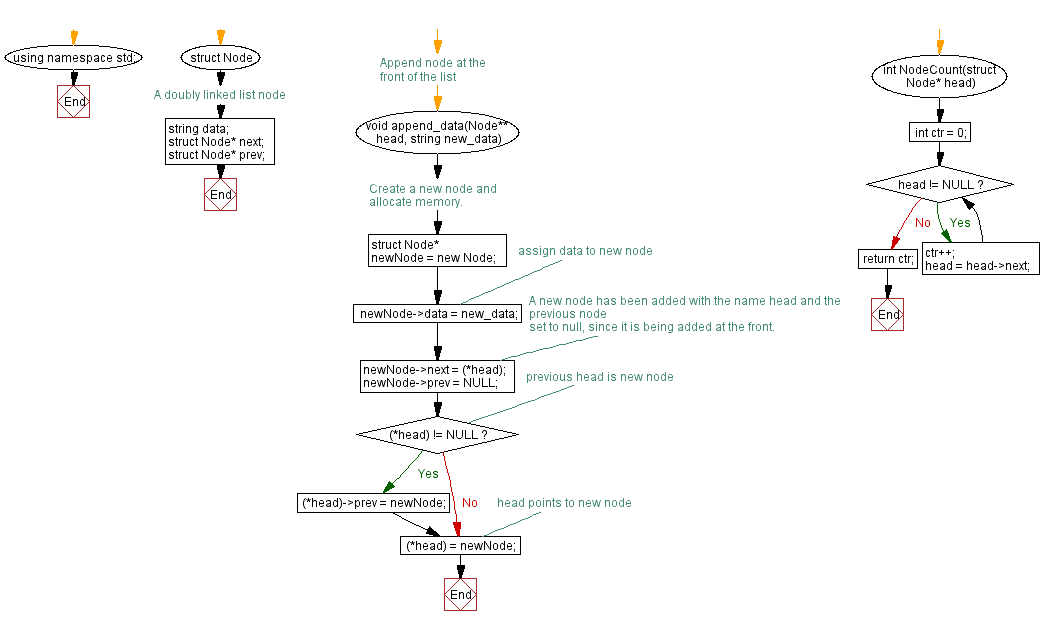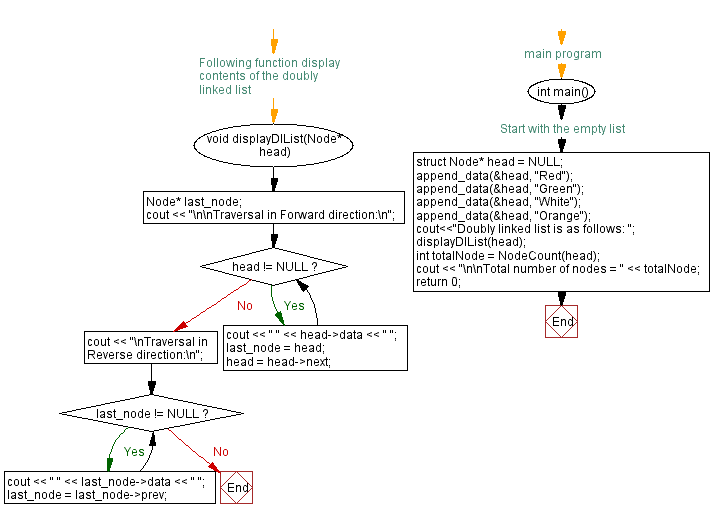﻿ C++ - Count number of nodes in a doubly linked list

# C++ Linked List Exercises: Count number of nodes in a doubly linked list

## C++ Linked List: Exercise-17 with Solution

Write a program in C++ to create a doubly linked list of n nodes and count the number of nodes.

Test Data:
Doubly linked list is as follows:
Traversal in Forward direction:
Orange White Green Red
Traversal in Reverse direction:
Red Green White Orange
Total number of nodes = 4

Sample Solution:

C++ Code:

``````#include <iostream>

using namespace std;

// A doubly linked list node
struct Node {
string data;
struct Node* next;
struct Node* prev;
};

//Append node at the front of the list
{
//Create a new node and allocate memory.
struct Node* newNode = new Node;

//assign data to new node
newNode->data = new_data;

// A new node has been added with the name head and the previous node
// set to null, since it is being added at the front.
newNode->prev = NULL;

}

{
int ctr = 0;
ctr++;
}
return ctr;
}

// Following function display contents of the doubly linked list
{
Node* last_node;
cout << "\n\nTraversal in Forward direction:\n";
cout << " " << head->data << " ";
}
cout << "\nTraversal in Reverse direction:\n";
while (last_node != NULL) {
cout << " " << last_node->data << " ";
last_node = last_node->prev;
}
}

//main program
int main() {
cout<<"Doubly linked list is as follows: ";
cout << "\n\nTotal number of nodes = " << totalNode;
return 0;
}
``````

Sample Output:

```Doubly linked list is as follows:

Traversal in Forward direction:
Orange  White  Green  Red
Traversal in Reverse direction:
Red  Green  White  Orange

Total number of nodes = 4
```

Flowchart:CPP Code Editor:

Previous C++ Exercise: Reverse doubly linked list.
Next C++ Exercise: Insert a node at the beginning of a Doubly Linked List.

What is the difficulty level of this exercise?

﻿

## C++ Programming: Tips of the Day

C++: const reference, before vs after type-specifier

No difference as const is read right-to-left with respect to the &, so both represent a reference to an immutable Fred instance.

Fred&const would mean the reference itself is immutable, which is redundant; when dealing with const pointers both Fred const* and Fred* const are valid but different.

It's a matter of style, but using const as a suffix since it can be applied consistently including const member functions.

Ref: https://bit.ly/3NmkTS7

We are closing our Disqus commenting system for some maintenanace issues. You may write to us at reach[at]yahoo[dot]com or visit us at Facebook